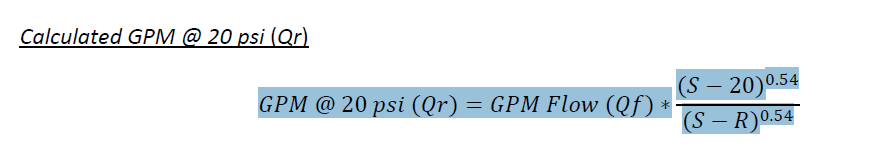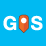# Subtraction in formula

122
1
06-01-2020 11:36 AMNew Contributor III

I am currently attempting to create a field based on a mathematical formula but the formula is getting held up do to the subtraction sign. I know this because I have performed calculations on other parts of the formula with no issue. The formula is shown below and the part I believe is causing issues is in bold. Thanks in advance.

\${gpm_flow} * pow(\${flwhyd_static_pressure_psi}-20,0.54) div pow(\${flwhyd_static_pressure_psi},0.54 - pow(\${reshyd_pressure_psi}, 0.54)

The formula I am attempting isby
Occasional Contributor III

Hi Jason,

try something like this:

\${gpm_flow} * (pow(\${flwhyd_static_pressure_psi}-20,0.54)/pow(\${flwhyd_static_pressure_psi}-\${reshyd_pressure_psi}, 0.54))

Cheers

Stefano